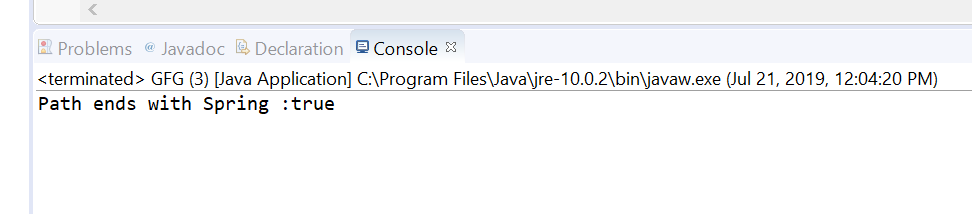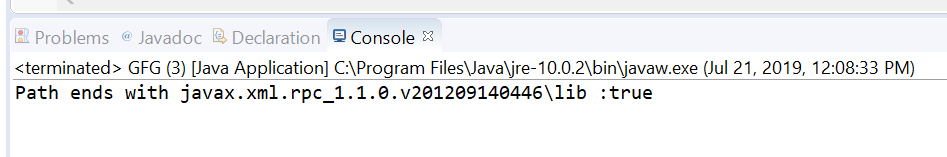Related Articles
Path endsWith() method in Java with Examples
• Last Updated : 23 Jul, 2019

endswith() method of java.nio.file.Path usec to check if this path object ends with the given path or string which we passed as parameter.
There are two types of endsWith() methods.

1. endsWith(String other) method of java.nio.file.Path used to check if this path ends with a Path, constructed by converting the given path string which we passed as a parameter to this method. For example, this path “dir1/file1” ends with “dir1/file1” and “file1”. It does not end with “1” or “/file1”. Note that trailing separators are not taken into account, and so invoking this method on the Path”dir1/file1″ with the String “file1/” returns true.

Syntax:

```default boolean endsWith(String other)
```

Parameters: This method accepts a single parameter other which is the given path string.

Return value: This method returns true if this path ends with the given path; otherwise false.

Exception: This method throws InvalidPathException If the path string cannot be converted to a Path.

Below programs illustrate endsWith(String other) method:
Program 1:

 `// Java program to demonstrate ` `// Path.endsWith(String other) method ` ` `  `import` `java.nio.file.Path; ` `import` `java.nio.file.Paths; ` `public` `class` `GFG { ` `    ``public` `static` `void` `main(String[] args) ` `    ``{ ` ` `  `        ``// create object of Path ` `        ``Path path = Paths.get(``"\\temp\\Spring"``); ` ` `  `        ``// create a string object ` `        ``String passedPath = ``"Spring"``; ` ` `  `        ``// call endsWith() to check path object ` `        ``// ends with passedPath or not ` `        ``boolean` `check = path.endsWith(passedPath); ` ` `  `        ``// print result ` `        ``System.out.println(``"Path ends with "` `                           ``+ passedPath + ``" :"` `                           ``+ check); ` `    ``} ` `} `

Output:2. endsWith(Path other) method of java.nio.file.Path used to check if this path ends with the given path as parameter to method or not.This method return true if this path ends with the given path; otherwise false.
If the passed path has N elements, and no root component and this path have N or more elements, then this path ends with the given path if the last N elements of each path, starting at the element farthest from the root, are equal.
If the passed path has a root component then this path ends with the given path if the root component of this path ends with the root component of the given path, and the corresponding elements of both paths are equal. Whether or not the root component of this path ends with the root component of the given path is filesystem-specific. If this path does not have a root component and the given path has a root component then this path does not end with the given path.
If the given path is associated with a different FileSystem to this path then false is returned.

Syntax:

```boolean endsWith(Path other)
```

Parameters: This method accepts a single parameter other which is the given path.

Return value: This method return true if this path ends with the given path; otherwise false.

Below programs illustrate endsWith(Path other) method:
Program 1:

 `// Java program to demonstrate ` `// java.nio.file.Path.(Path other) method ` ` `  `import` `java.nio.file.Path; ` `import` `java.nio.file.Paths; ` `public` `class` `GFG { ` `    ``public` `static` `void` `main(String[] args) ` `    ``{ ` ` `  `        ``// create an object of Path ` `        ``Path path ` `            ``= Paths.get(``"D:\\eclipse"` `                        ``+ ``"\\plugins"` `                        ``+ ``"\\javax.xml.rpc_1.1.0.v201209140446"` `                        ``+ ``"\\lib"``); ` ` `  `        ``// create a path object which we will pass ` `        ``// to endsWith method to check functionality ` `        ``// of endsWith(Path other) method ` `        ``Path passedPath = Paths.get( ` `            ``"javax.xml.rpc_1.1.0.v201209140446"` `            ``+ ``"\\lib"``); ` ` `  `        ``// call endsWith() to check path object ` `        ``// ends with passedPath or not ` `        ``boolean` `check = path.endsWith(passedPath); ` ` `  `        ``// print result ` `        ``System.out.println(``"Path ends with "` `                           ``+ passedPath + ``" :"` `                           ``+ check); ` `    ``} ` `} `

Output:References:

Attention reader! Don’t stop learning now. Get hold of all the important Java Foundation and Collections concepts with the Fundamentals of Java and Java Collections Course at a student-friendly price and become industry ready.

My Personal Notes arrow_drop_up
Recommended Articles
Page :